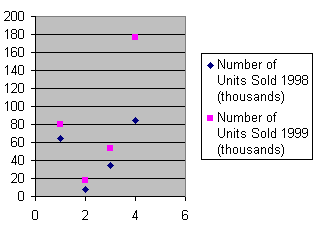scatter diagram

(redirected from Dot chart)
Also found in: Dictionary, Medical.

scatter diagram

(petrology)
(statistics)
A plot of the pairs of values of two variates in rectangular coordinates. Also known as scatter gram.

or

scattergram

a diagram produced on a graph to illustrate the relationship between two variables by indicating the values of these for each case in the sample. It may be a first stage in data analysis, allowing ‘eye-balling’ of the data to observe any association or CORRELATION between the variables.

scatter diagram

A graph plotted with dots or some other symbol at each data point. Also called a "scatter plot" or "dot chart."

A Scatter DiagramSite: Follow: Share:
Open / Close